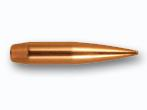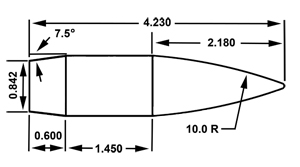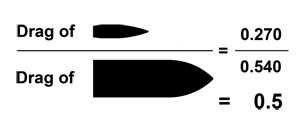••••Close×

## It started back around 1850 when ballisticians of many countries began experiments in an effort to improve the accuracy of artillery shells and the measurements of the drag or air resistance they encountered during flight. Basically, the necessities of war meant everyone wanted more accuracy.

There were no computers back in those days so all the mathematical solutions to these very complex equations were hand written. These took months and even years to complete. Between 1875 and 1898, German, French, Russian and English ballisticians worked feverishly to quantify air drag resistance of artillery shells and finally came up with a standard model of projectile on which further calculations could be based.

This made it a little easier to calculate the trajectories of new shaped projectiles by reducing the time required for new calculations. This standard reference projectile shape is known as the G1 Standard bullet. "G" stands for the Gâvre Commission of the French Naval Artillery. This commission conducted many air resistance firings at the Gâvre Proving Ground utilising a Belgian chronograph manufactured in 1864. Figure 1  illustrates the shape and measurement of this projectile in "calibres". One calibre is the width of the projectile.The G1 projectile was one pound in weight (lead), and was one inch in diameter. This hefty 7000 grain (1lb) projectile is basically what most small-arms projectiles today are measured against for their ballistic coefficient reference numbers.

Between the First and the Second World Wars, again, out of necessity, further projectile shapes were designed, calculated and mapped for air drag resistance. Different shapes emerged, with angled bases called "boattails" and pointed tips. These tips were constructed by making the radius of the curve at the front of the projectile – called the ogive – larger. The ogive radius of the Type 1 projectile in Figure 1 is 2.00 calibres. The radii of the new projectile types were now between 6 and 10 calibres.

These projectiles were numbered G2, G3, G5, G6, G7 and G8. They ranged from flat based with more rounded noses to classic spitzer shaped projectiles with angled boattails, as seen in today's long-range small arms ammunition.

There are many projectiles we use today that closely match these reference models. For instance, the .30-cal Berger 210gn VLD very closely resembles the G7 model, although it is not actually a G7. The .30-cal Nosler 150gn Ballistic Tip very closely resembles the G6.

The exact model of the G7 projectile is illustrated in Figure 2. If another projectile has all the same measurements except that the boattail angle is 8° instead of 7.5°, it is not a G7.Drag and Form Factors

The amount of drag that a projectile experiences in supersonic flight depends heavily on its shape and velocity. The speed of sound at sea level at a 15°C and 78% relative humidity (RH) may be around 1116fps (340m/s). This can be referred to as Mach 1. A projectile travelling at this speed is travelling at the same speed that sound travels in the same atmosphere. A projectile travelling in this same atmosphere at 2232fps will be doing Mach 2. Mach 2.5 would be approximately 2790fps and so on.

A projectile travelling at these speeds has shock waves of compressed air attached to the front and rear, which tend to draw a large amount of energy from it, thus slowing it down aggressively. These shock waves are attached to the projectile at certain angles that change at different speeds and, as a result, draw different amounts of energy from the projectile. What this means is that the amount of drag or resistance on the projectile varies at different speeds.

The two main factors that affect drag (air resistance) on a spin-stabilised free-flight projectile are shape and velocity.

The blunt-nosed G1 projectile will be less efficient through the air than the G7 as it is simply not as streamlined. The relationship of a projectile's weight and its cross-sectional area is called the "sectional density". An example that may shed some light on this can be explained with arrows and crossbow bolts.

If an arrow made with an aluminium shaft, a broadhead tip and three plastic flights is fired from a bow at a speed of 380fps, it will only travel so far before hitting the ground. If a crossbow bolt made of the same width aluminium shaft but only a third of the length, the same broadhead tip and plastic flights is fired at the same velocity of 380fps, it would hit the ground earlier. Why? It has the same frontal area exposed to the oncoming airflow but it is lighter due to being shorter. Projectiles of the same calibre, but different weights, have different sectional densities. The crossbow bolt has a lower sectional density than the arrow.

However, this crossbow bolt that has a lower sectional density will travel further if launched at a higher velocity. This is usually the case with crossbows anyway.

The "form factor" of a projectile is a numerical figure that compares a projectile's unique drag to that of a standard or reference bullet such as the G1 or G7 projectile. The lower the form factor (FF) of the projectile, the more efficient it is. Let's compare the G1 to the G7.

At a velocity of 2792fps the amount of drag on the G1 projectile can be quantified into a numerical figure of, say, 0.540. At the same velocity, the G7 projectile may have a drag coefficient of 0.270. The lower the drag coefficient (CD), the more efficient it is through the air. Compare these two by dividing the G7 by the G1 figures and you have the G1 form factor of 0.5. If the figure is below 1.0 the projectile is more efficient than the reference projectile.In Figure 3 above you can see that the G7 is twice as efficient in air when compared to the G1.

Ballistic Coefficients

A ballistic coefficient is a numerical figure usually between 0 and 1 that allows you to see basically how well it penetrates through the air. The closer, more accurate description would be "a numerical factor that describes the rate of velocity degradation of a particular projectile when compared with the rate of velocity degradation of a standard projectile".

This figure is determined by two attributes of the projectile: sectional density and form factor.

The sectional density of a 210gn Berger VLD would be as follows:

 Sectional Density (SD) = Bullet weight in pounds (lb) Bullet calibre x Bullet calibre

OR

 Sectional Density (SD) = Weight in grains (210) ÷ 7000 (lb) .308 x .308 = 0.316

Divide this number by the G1 form factor (i1), and you have the G1 ballistic coefficient.

 BC = SD i

SD = Sectional Density; i = Form Factor

Therefore:

 BC = 0.316 0.489 (i1 form factor) G1 BC = 0.646 (at 2790fps only)

If this projectile was travelling at a lower velocity, the G1 ballistic coefficient would change. At 2000fps the FF may be around .496. This would mean the G1 BC would be:

 G1 BC = 0.316 0.496 (i1 form factor) G1 BC = 0.637 (at 2000fps only)

And again at 1500fps:

 G1 BC = 0.316 0.532 (i1 form factor) G1 BC = 0.593 (at 1500fps only)

You can see what is happening here. The i1 FF is changing at different speeds because it is made from the drag coefficient. The drag coefficient is changing as the velocity is changing (slowing down). The G1 BC given to us by Berger is the average coefficient experienced throughout the entire supersonic flight of the projectile. In this instance, the average G1 BC of this Berger 210gn projectile is 0.631. BCs supplied by other manufacturers may not be the average, but ones tested at short range at one or more velocities.

BC MYTHS AND FACTS

1. BCs are calculated all the same way

No, they aren't. They are calculated with different measuring equipment, some using ICAO standard atmospheres and other using Army Std Metro Atmospheres. Some are measured close to the chronographs and others are measured at some distance away. Some lower-velocity BCs are measured with down-loaded cartridges at close range.

2. G1 BCs change with velocity

Yes, they do. As described earlier, as velocity changes, so does the drag and therefore the form factor. Change the FF and the G1 BC changes.

3. G7 BCs don't change with velocity

Yes, they do. Only an exact G7 projectile will not, as the G7 BC of a G7 projectile is comparing it against itself. Other projectiles that are close to the G7 profile will have a different form factor from the G7 value of 1.0. As these other projectiles' form factors (i7) change with velocity, so do the BCs, just to a lesser degree than G1s.

4. All form factors are the same

No, they are not. The FF to calculate a G1 BC must be a G1 form factor, represented as i1.

A G7 BC needs a G7 FF represented as i7. The FF numerical value of a G1 projectile equals 1.0. The FF numerical value of a G7 projectile equals 1.0. The G7 FF of a .30-cal 210gn Berger VLD may be 0.983, showing it is a little more efficient than the G7 standard projectile. The G7 BC of this would then be:

 G7 BC = 0.316 (SD) 0.983 (i7) = 0.321

5. BCs are not important

Yes, they are. Without this knowledge we cannot estimate the loss of velocity down range. Knowing the muzzle velocity is great but knowing the terminal velocity just before the projectile strikes the target in a hunting situation is very helpful. This can educate us as to what projectiles will perform on the game we choose to take.

6. Sectional density information is helpful

Yes, it is. Especially when hunting dangerous game. When taking dangerous game, the projectiles should have a sectional density of over 0.300. This gives the professional hunting guide (PH) vital information on the penetration capabilities of the projectile.

Summary

Modelling drag on free-flight spin-stabilised projectiles has been going on for a long time now. Born out of necessity as a result of war, these calculations are very complicated and therefore much misunderstood by the sporting shooter and hunter.

Some of us only require small amounts of this information and others, a fair bit more. There is a lot of information available today, which can be highly attributed to the speed at which information travels. With the massive steps forward in technology in the last 20 years, information such as this is now required by some sporting shooters, particularly those in the long-range shooting community.

Three simple areas should be remembered:

1. The higher the ballistic coefficient, the better it slices through the air

2. The higher the drag coefficient, the worse it slices through the air

3. The higher the sectional density, the deeper the penetration

The term ballistic coefficient is a very loose one in itself, and its use begs the question, what type of ballistic coefficient? The term, when understood a little better, can help you as a shooter make better informed decisions when choosing projectiles in the future.

Glen Roberts is the chief instructor of Precision Shooting Australia : http://www.precisionshooting.com.au